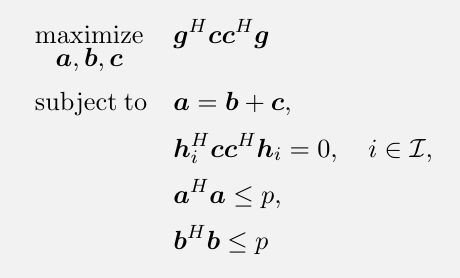# Lagrangian method for complex-valued vector variables of an optimization problem

Can I use the Lagrange multipliers method for the complex-valued vector variables? or should I rewrite all the constraints for real-valued vector variables?
for example, in the following problem, should I rewrite all the constraints for real-valued vector variables?CVX does accept complex-valued equality constraints. which are interpreted as applying separately for each of real and imaginary part.

CVX does not accept complex-valued inequality constraints, But you can write then as separate constraints for each of real and imaginary part.

An inequality constraint such as h`'*c*c'*h <= 0` (of course your constraint is an equality constraint) could potentially trigger an error message in CVX due to roundoff-level imaginary term, even though the imaginary part would be zero if it were computed in exact arithmetic. That can be obviated either by applying `real(...)` or by rewriting with parentheses or intermediate expressions: for instance `(c'*h)'*(c'*h) == 0`, or by `x = c'*h, x'*x == 0`

As for `a == b + c`. The best way is probably to declare `b` and `c` as variables, and then include the (expression assignment) statement `a = b + c;` (note the single `=`) prior to use of `a` in constraints.

Edit: I have corrected the first three paragraphs. I had a “writeo” and interchanged which of equality and inequality constraints CVX allows to be complex-valued.

1 Like